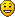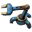## Booleans!

This board is to show, discuss and archive useful combinator- and logic-creations.
Smart triggering, counters and sensors, useful circuitry, switching as an art, computers.
piriform
Fast InserterPosts: 117
Joined: Mon Jan 25, 2016 10:02 pm
Contact:

### Booleans!

A few days ago I had a thought that it might be interesting to do some Boolean operations within Factorio.
At a minimum I was hoping to be able to perform AND, OR, NOT and XOR on at least two items. Not knowing any magical, mystical ways to fool the combinators, I had to do it the hard way.

Here is a picture of the resulting circuit:

Description of the blocks and circuits

Yellow rectangle outlines the 8 bit binary decoder. For an explanation please refer to DaveMw's reply in my original post ("Binary decoder").

Red rectangle outlines the register that stores and adds the two numbers. Red signal clears it out and the two numbers arrive in succession and are added. This implies that each bit can have two values 1 or 2 (0 is implied by the absence).

Operator logic (i.e. OR,AND,NOT,XOR) is outlined by the blue rectangle. It has 4 rows, starting from the top:

OR logic. : ea>0;ea=1,ea+0;ea,
AND logic. : ea>1;ea=1,ea+0;ea, (if the bits are same in both words the value of the bit will be 2)
XOR logic. : ea=1;ea=1,ea+0;ea, (if the bits are different in both words the value of the bits will be 1)
NOT logic. :ea >0;ea-1;ea*-1;ea, (sums the input from register and negative bits from the constant combinator next to it before subjecting the bits to the test)
The adders in the first 3 rows performing ea+0;ea delay the signal.

Operation select and conversion back to decimal is outlined by the green rectangle
The 4 combinators select the desired operation (coming in from the black rectangle circuit)
OR : copper ore >0; ev=count
AND : stone >0; ev=count
XOR : copper plate>0; ev=count
NOT: wood >0; ev=count
The column of adders convert the bits back to the decimal by multiplying each bit with appropriate power (i,e, 2^(n-1)) and summing the results.

The circuitry in the fuchsia (??) rectangle generates test signals and is not strictly necessary (although some form of clearing and 1 tick wide word generation IS)

Discussion of result
Size
8 bit unit, as shown here, is reasonably compact -24 for binary decoder, 1- register, 8 logic, 4 selector, 8 decimal encoder. Total = 45 +1 constant
16 bit unit would require additional 32 combinators (total 77+1)
Correctness
I tested it a fair bit and it appears to be correct. If you DO find any errors, please let me know.

Speed
The total propagation delay is 3+1+2+2=8 ticks (assuming register takes 1 tick, although I have a suspicion it's 0. If anyone can clarify I'd be grateful).
But, a new set of words can be clocked every 3 ticks, so pipelining is possible (20 ops!).

Why and where in the World would you want to use this contraption?
Here are some ideas
-whenever you want to activate multiple devices at once (use just the decoder and send one word-no need for fancy timing)
-whenever you are monitoring multiple devices or conditions and want to ignore some ( mask out the desired bits using AND word )
-whenever you want to monitor change in status of several signals (XOR them)
-design a binary computerIf you do have any comments or suggestions, I'd like to hear from you.

Blueprint string